# Teaching Multiplication Facts: How to Use Multiplication Patterns to Help Students Learn

Page content

Students are often overwhelmed by the thought of learning and memorizing their multiplication facts. At first glance, the standard multiplication table appears to be a jumble of problems with little rhyme or reason. For your more reluctant learners, this can translate into feelings of panic, inadequacy or even resentment. The key to successfully teaching multiplication facts, therefore, is breaking the task into manageable lessons. Identifying and using multiplication patterns does just that!

## Learning Patterns Means Only Having to Memorize 10 Facts

Learning multiplication facts does not seem as daunting when you tell your students they will only have to memorize a total of 10 facts! How is this possible, they might ask? The answer is multiplication patterns.

From very early on, children have been taught to identify patterns - in reading, in spelling, in art, in music and of course, in mathematics. The Multiplication Table is no exception. It is made almost entirely of repeating patterns. Once these patterns are identified and understood, it can be noted that there are only 10 remaining multiplication facts that do not fit a specific pattern. For these problems, the only available learning tool is memorization. Still…the thought of having to memorize 10 problems is much less overwhelming than the thought of memorizing an entire table!

## Begin with a Multiplication Book

Having students create their own multiplication “books” is a great way to begin. Provide each student with two pieces of construction paper and eight sheets of manuscript paper. This will serve as the basis for your book. The title of the book is My Multiplication Book. The first page of the book should define the word “multiplication”:

What is multiplication?

After eliciting responses from your students, you should (together) construct a definition that reads something like this:

Multiplication is a fast way to add when joining groups of equal size.

3 x 4 = 12 (How many groups x How many in each group = How many all together)

Have your students write this definition on the first page of their multiplication book.

The next step is to explain the Commutative Property of Multiplication:

When two numbers are multiplied together, the product is the same regardless of the order of the multiplicands. For example 3 x 2 = 2 x 3.

By understanding this principle, students see that many problems can be overlooked because they are actually duplicates. Have them write this definition on the second page of their book.

The following pages of the book will cover the fact families that show definitive patterns, the associated rules, the numerical sentences that make up the fact family and a pictorial representation of the Commutative Property of Multiplication.

Below you will find examples of each of these pages.

## My Multiplication Book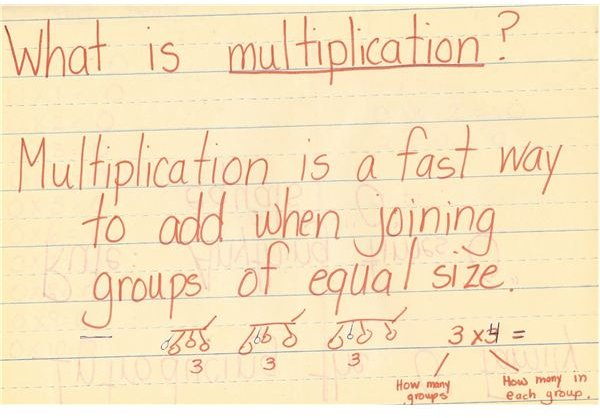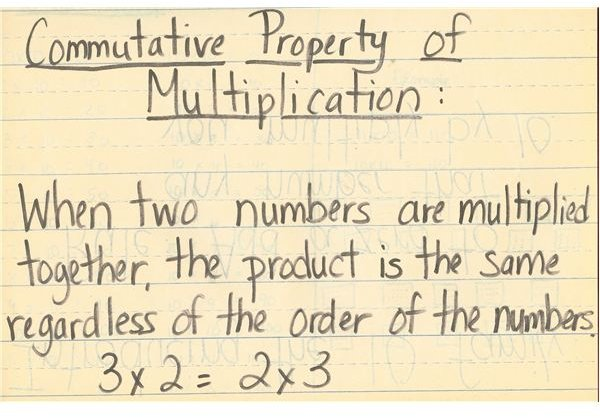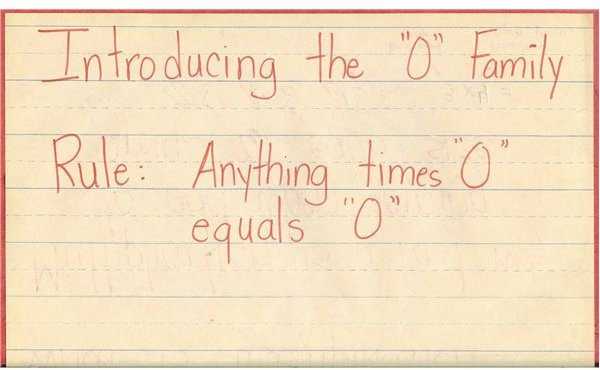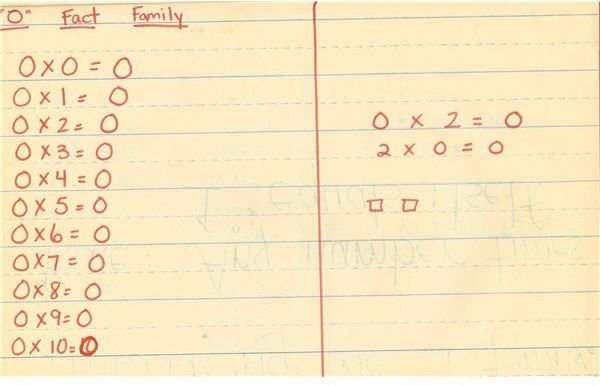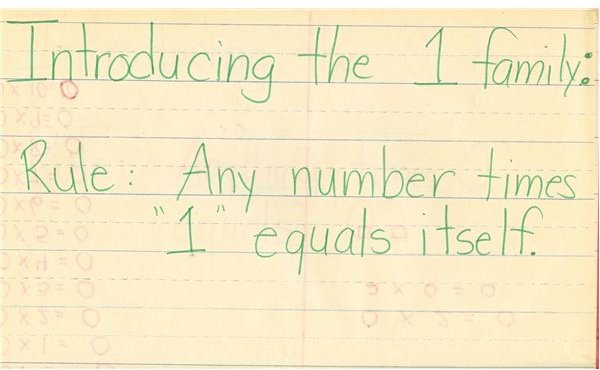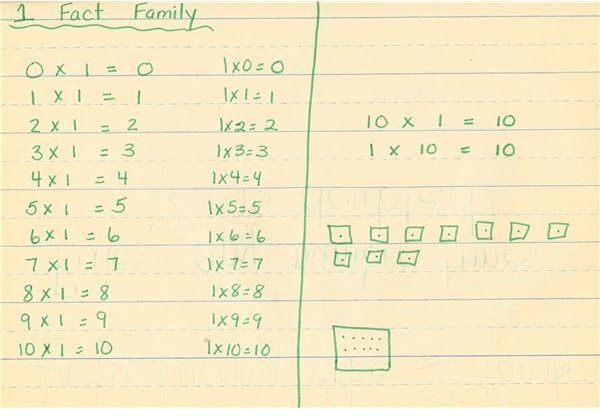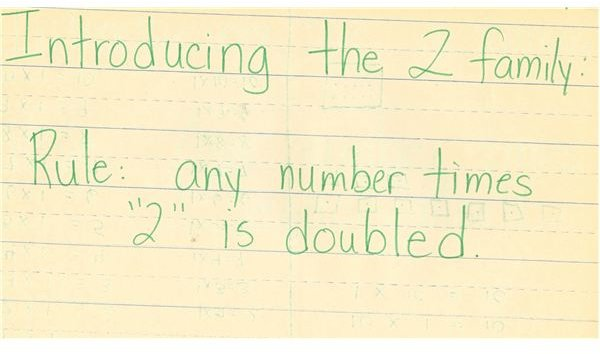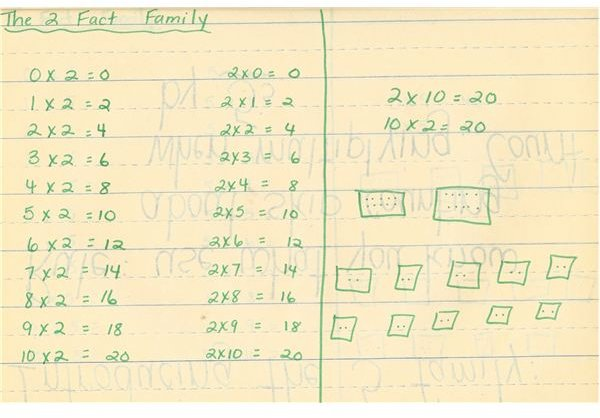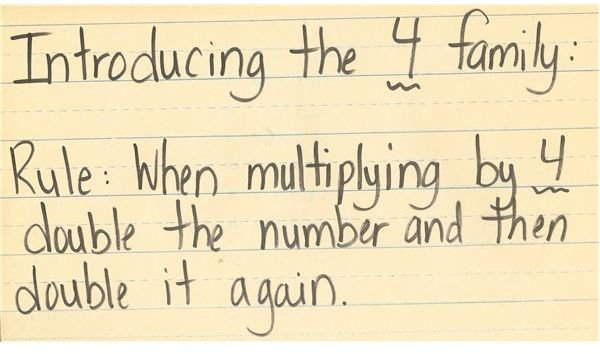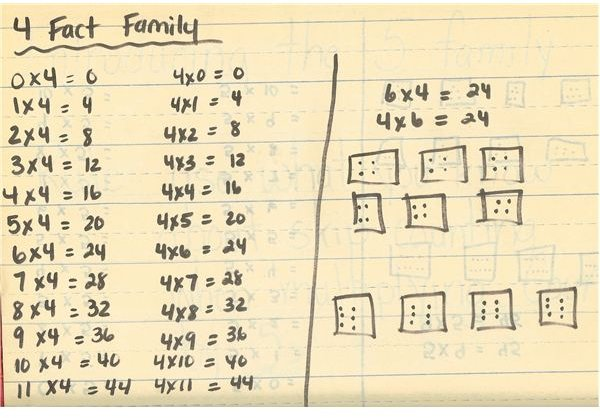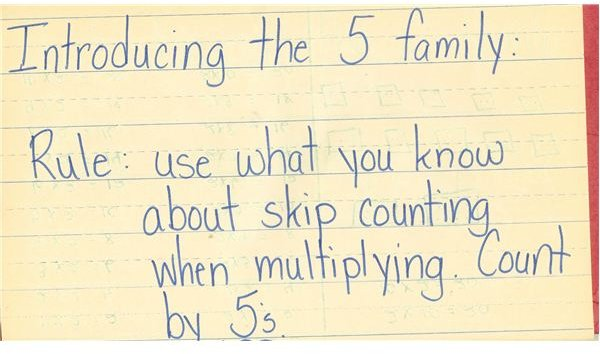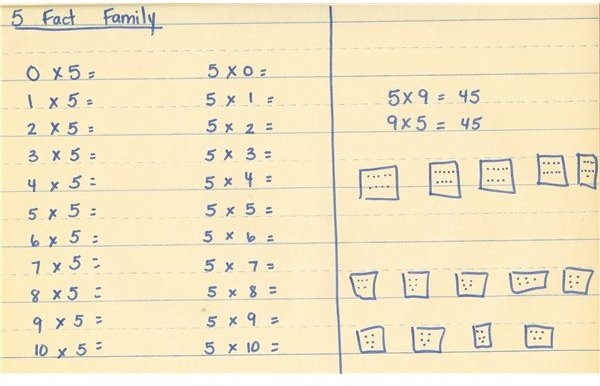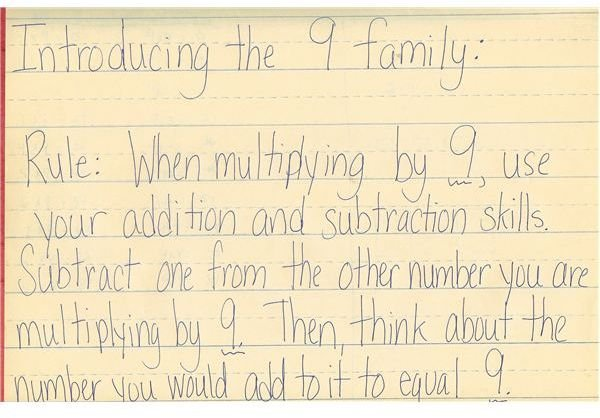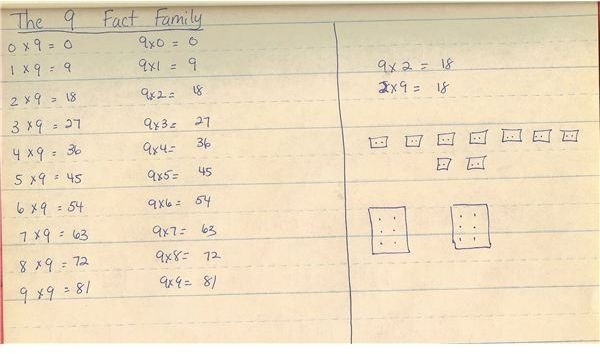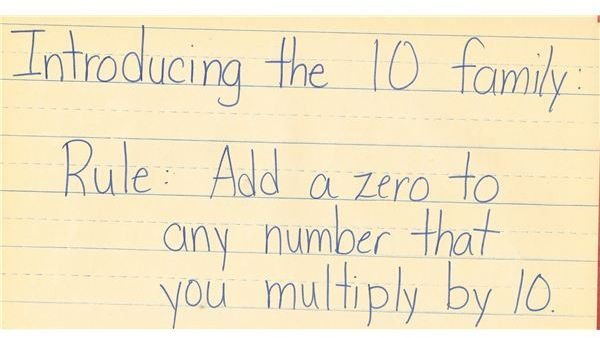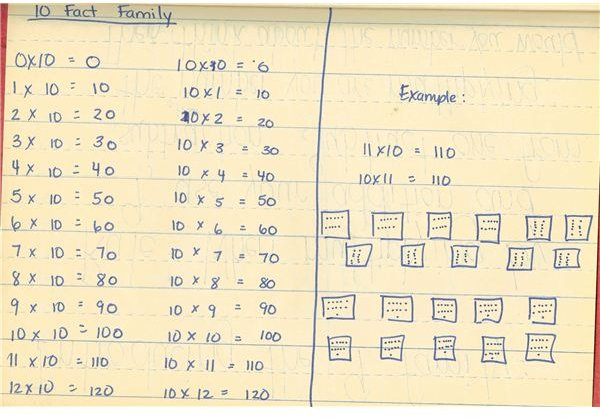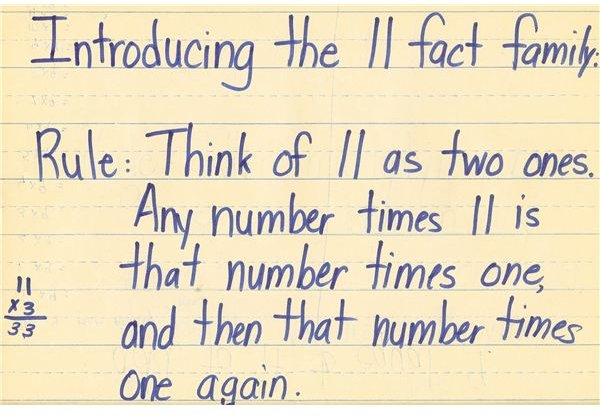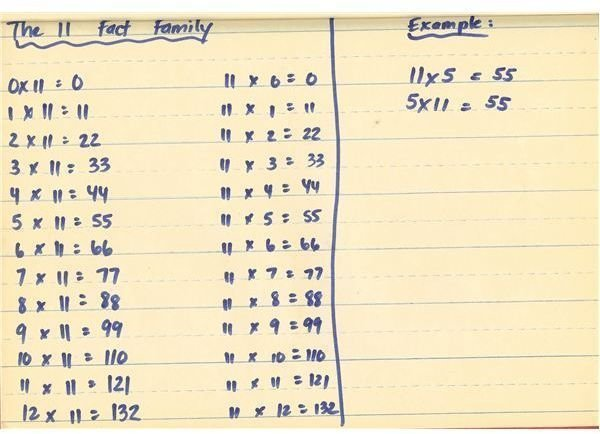## Using a Multiplication Table as a Visual Aid

An effective way to manage the lessons is to introduce one Rule/Family each day. Using a large Multiplication Table can be a terrific visual aid. As you learn each Fact Family, cross them off on the Multiplication Table. That way, your students can actually see the progress they are making in learning the multiplication facts:

## Multiplication Table after the 1’s Family is studied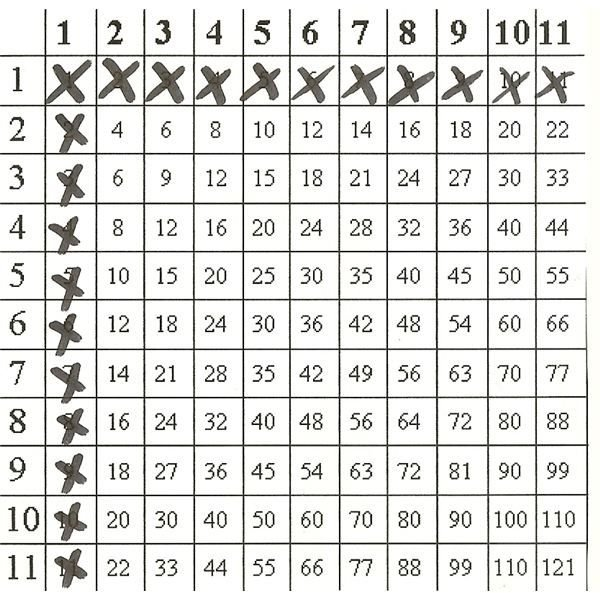## Only 10 Problems Left

The last page of your Multiplication Book will include the 10 multiplication problems that are not covered by the Fact Family patterns studied:

3x3, 3x6, 3x7, 3x8, 6x6, 6x7, 6x8, 7x7, 7x8 & 8x8

(Remember…due to the Commutative Property of Multiplication, there are only 10 problems instead of 20.)

This is how the Multiplication Table you are using for demonstration will look when you have completed teaching multiplication facts. (The circled problems are the 10 that must be memorized):

## Multiplication Table at Completion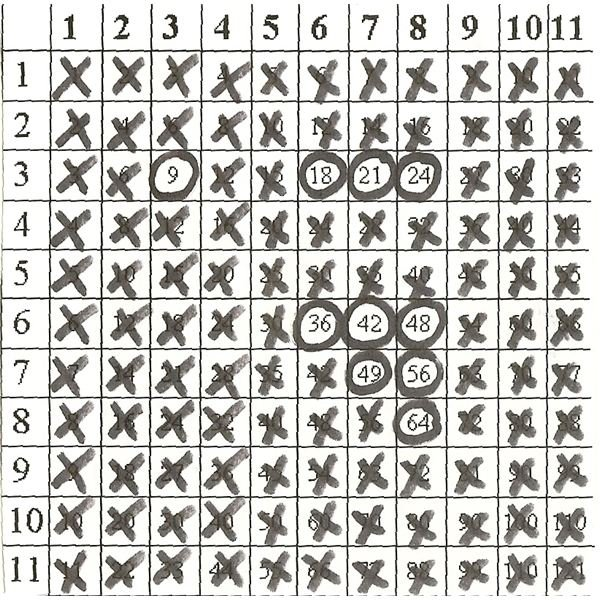• The activities and images offered in this article are the author’s own